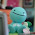## Sunday, January 16, 2011

### Paulo's Percent Review Video

A percent means out of 100.
Ex: 50% means 50/100, 50 out of 100, or 0.50

4.1Representing Percent

Representing percents means showing a percent as a picture in a hundred grid.
Ex. 50% on a hundred, 50 squares are shaded in a hundred grid so it would be 50%.

4.2 Fraction,Decimal,and Percents

Fractions,decimals, and percents can be used to represent numbers in various situations
-Percents can be written as fractions and as decimals.
Ex. 1/2%=0.50%, 0.5/1000=o.005, 150%=150/100=1.5 or 1 1/2

4.3 Percent of a number

-You can use mental math strategies such as halving,doubling, and dividing by ten to find the percents of some numbers.
-To calculate the percent of a number,write the percent as a decimal and then multiply by the number.
Ex. 12 1/2% of 50=0.125 x 50=6.25 ( 12 1/2 %=12.5%=0.125)

4.4 Combining Percent

-Percent can be combined by adding to solve problems. 5% + 7%=12%
-To calculate the increase in a number.
Ex. 12% of 100= 0.12 x 100=12,100 + 12=112
-You can multiply the original number by a single percent greater than 100
Ex. 112% of 100=112 x 100 = 112

Percent of percents can be used to determine amounts that result from consecutive increases or decreases.

This is my percent preview video:

#### 1 comment:

1.Good Job Paulo, you should add colour to it so it would pop out more. Also you should you use only bolded for the important parts not the whole text. Other than that, great jojb Paulo! Keep up the good work!